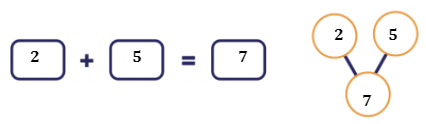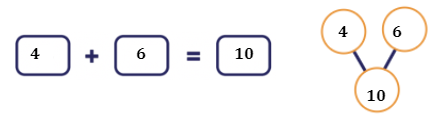Study P1 Mathematics Addition within 10 - Geniebook1. Addition within 10 by number bonds
2. Addition within 10 by counting on

## Topic Recap

Before we proceed, let’s do a recap of Numbers to 10. So far, we have learnt:

• How to read and write numbers in numerals and words
• Comparing the number of items in given sets

Let’s see if you remember these concepts well!

## Read And Write Numbers In Numerals And Words

Question 1:

Fill in the numerals.

WORDS NUMERALS
Five
Nine
One
Three
Ten
Seven
Six
Two
Four
Eight
Zero

WORDS NUMERALS
Five 5
Nine 9
One 1
Three 3
Ten 10
Seven 7
Six 6
Two 2
Four 4
Eight 8
Zero 0

## Comparing Numbers

Question 2:

Let’s match and compare.When we match each spoon to a plate, we see that there are some plates left. When you count both items, the number of spoons is smaller than the number of plates.We can say that:

There are more plates than spoons.

There are fewer spoons than plates.

## 1. Addition Within 10 By Number Bonds

Let’s do a quick recap on number bonds.

Tom and Jerry were given 5 doughnuts each.2 and 3 are the parts and 5 is the whole. Tom has 5 doughnuts altogether.1 and 4 are the parts and 5 is the whole. Jerry has 5 doughnuts altogether.

Question 1:

Terry has 2 boxes of muffins. There are 3 strawberry muffins and 4 chocolate muffins. How many muffins does Terry have altogether?7

Explanation:$$3 + 4 = 7$$

Question 2:

There are 2 boys and 4 girls. Which of the number bonds shows the total number of children correctly?(Hint: Can you identify the parts? In the question, total refers to WHOLE. Do remember to identify the two parts and the whole in every option.)

(2)

Explanation:

Let’s count each part first.The parts will be 2 boys and 4 girls.

Therefore, the total number of children will be $$2 + 4 = 6$$.

Question 3:

There are 3 blue flowers and 7 yellow flowers. How many flowers are there altogether?1. 4
2. 7
3. 3
4. 10

(4) 10

Explanation:

The two parts given are 3 blue flowers and 7 yellow flowers3 and 7 are the parts. To find the whole, we will use $$3 + 7 = 10$$

## 2. Addition Within 10 By Counting On

Previously, we were adding numbers using number bonds. The next Math concept that we are going to learn is addition by counting on.

Example:

Reanne has 2 boxes of muffins. There are 5 strawberry muffins and 3 chocolate muffins. How many muffins does Reanne have altogether ?Can you tell me which are the parts? Our parts will be 5 and 3.

We count on from the greater number.After 5 strawberry muffins, we can continue counting on to 6, 7 and 8.

We can also say that:

$$5 + 3 = 8$$

We can use either of the two methods (number bonds or counting on) to add two numbers. To be sure, we can use one of the methods and check again using the other method.

Question 1:

There are 7 purple pencils and 2 blue pencils. How many pencils are there in total ?1. 5
2. 8
3. 9
4. 10

(3) 9

Explanation:

Start counting on from the purple pencils. There are 7 purple pencils.The total number of pencils is 9.

You can use the number bonds method below to check your answer too.Question 2:

How many ice-creams are there in total?1. 7
2. 8
3. 9
4. 4

(2) 8

Explanation:

In this question, you are asked to find the whole. The parts given are 4 and 4.

If we count on, we will get…The whole will be 8.

Check using the number bonds.Question 3:

How many pies are there below in total?1. 5
2. 2
3. 3
4. 6

(1) 5

Explanation:

The parts given are 2 and 3.Question 4:

Which of the following sentences best represents the diagram below?1. $$2 + 3 = 5$$
2. $$5 + 2 = 7$$
3. $$2 + 5 = 8$$
4. $$6 + 2 = 8$$

(4) 8

Explanation:

Let’s identify the parts and whole. Our parts will be 6 cats and 2 fish.Question 5:

Which of the following adds up to 6?

1. 4 and 3
2. 5 and 5
3. 6 and 1
4. 4 and 2

(4) 4 and 2

Explanation:

Add the parts given in each option.

1. $$4 + 3 = 7$$
2. $$5 + 5 = 10$$
3. $$6 + 1 = 7$$
4. $$4 + 2 = 6$$

Question 6:

Which of the following does not add up to 10 ?

1. 3 and 7
2. 8 and 2
3. 4 and 5
4. 0 and 10

(3) 4 and 5

Explanation:

Add the parts given in each option.

1. $$3 + 7 = 10$$
2. $$8 + 2 = 10$$
3. $$4 + 5 = 9$$
4. $$0 + 10 = 10$$

Question 7:

Which of the following has the same answer as $$5 + 4$$?

1. $$9 + 0$$
2. $$5 + 3$$
3. $$8 + 2$$
4. $$6 + 1$$

(1) $$9 + 0$$

Explanation:

$$5 + 4 = 9$$

Add the parts given in each option.

1. $$9 + 0 = 9$$
2. $$5 + 3 = 8$$
3. $$8 + 2 = 10$$
4. $$6 + 1 = 7$$

Question 8:

Which of the following has the smallest answer?

1. $$4 + 1$$
2. $$2 + 3$$
3. $$1 + 2$$
4. $$6 + 4$$

(3) $$1 + 2$$

Explanation:

Add the parts given in each option.

1. $$4 + 1 = 5$$
2. $$2 + 3 = 5$$
3. $$1 + 2 = 3$$
4. $$6 + 4 = 10$$

The smallest number is 3.

Question 9:

Which of the following has the greatest answer?

1. $$3 + 1$$
2. $$2 + 8$$
3. $$4 + 5$$
4. $$6 + 2$$

(2) $$2 + 8 = 10$$

Explanation:

Add the parts given in each option.

1. $$3 + 1 = 4$$
2. $$2 + 8 = 10$$
3. $$4 + 5 = 9$$
4. $$6 + 2 = 8$$

The greatest number is 10.

Question 10:

Which of the two buses add up to 8?

1. A and C
2. B and D
3. C and E
4. A and E

(4) A and E

Explanation:

Let’s find the total for all the options given.

1. $$\text{A and C} \qquad 5 + 4 = 9$$
2. $$\text{B and D} \qquad 8 + 1 = 9$$
3. $$\text{C and E} \qquad 4 + 3 = 7$$
4. $$\text{A and E} \qquad 5 + 3 = 8$$

## Challenge Yourself

Question 1:

Mavin has 2 apple pies. John has 5 apple pies. How many apple pies do Mavin and John have altogether ?1. 2
2. 5
3. 3
4. 7

(4) 7

Explanation:

When we add 2 and 5, we get 7.Question 2:

There are 4 white rabbits and 6 brown rabbits in a pet shop. How many rabbits are there in the pet shop in total?1. 2
2. 6
3. 10
4. 4

(3) 10

Explanation:

When we add 4 and 6, we get 10.Hope you had fun learning and solving these math problems!

• Addition within 10 by number bonds
• Addition within 10 by counting on

Continue Learning
Numbers To 10 Picture Graphs 1
Shapes 1 Ordinal Numbers
Subtraction Within 20 Addition Within 10
Addition And Subtraction Within 10 Numbers To 20
Addition Within 20 Numbers To 100PrimaryPrimary 1EnglishMaths
Numbers To 10
Picture Graphs 1
Shapes 1
Ordinal Numbers
Subtraction Within 20
Numbers To 20
Numbers To 100Primary 2Primary 3Primary 4Primary 5Primary 6SecondarySecondary 1Secondary 2Secondary 3Secondary 4